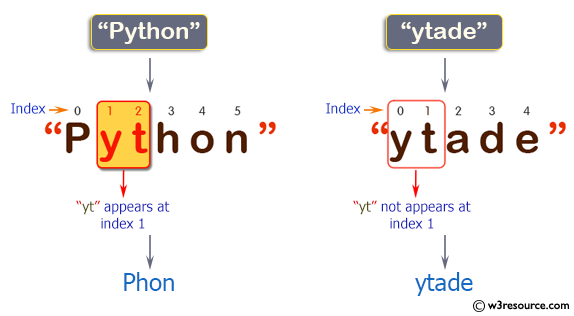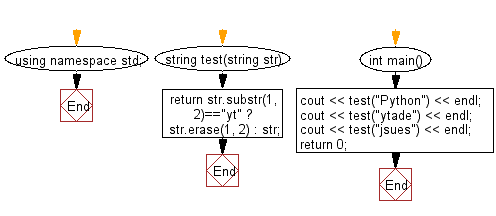﻿ C++ : Check if a text appears at index 1 in a given string# C++ Exercises: Check if a string 'yt' appears at index 1 in a given string

## C++ Basic Algorithm: Exercise-17 with Solution

Write a C++ program to check if a string 'yt' appears at index 1 in a given string. If it appears return a string without 'yt' otherwise return the original string.

Sample Solution:

C++ Code :

``````#include <iostream>
using namespace std;

string test(string str)
{
return str.substr(1, 2)=="yt" ? str.erase(1, 2) : str;
}

int main()
{
cout << test("Python") << endl;
cout << test("jsues") << endl;
return 0;
}
``````

Sample Output:

```Phon
jsues
```

Pictorial Presentation:Flowchart:C++ Code Editor: